Over a million developers have joined DZone.{{announcement.body}}
{{announcement.title}}

# Python Thread Tutorial (Part 1)

DZone 's Guide to

# Python Thread Tutorial (Part 1)

### In this tutorial, we take a look at how Python threads can be used to make code run smoother and faster, helping you garner insights from data quicker.

· Big Data Zone ·
Free Resource

Comment (3)

Save
{{ articles.views | formatCount}} Views

A Python thread is like a process, and may even be a process, depending on the Python thread system. In fact, Python threads are sometimes called “lightweight” processes, because threads occupy much less memory, and take less time to create than do processes.

Threads allow applications to perform multiple tasks at once. Multi-threading is important in many applications.

In Python, a threading module is used to create the threads. In order to create threads, threading modules can be used in two ways. First, by inheriting the class and second by the use of thread function.

## Python Thread Creation Using Class

Let us understand by using code.

``````import threading

class mythread(threading.Thread):

def __init__(self, i):

threading.Thread.__init__(self)

self.h = i

def run(self):

print“ Value send“, self.h

thread1 = mythread(1)

thread1.start()``````
• New class `mythread` inherits the Python `threading.Thread` class.
• `__init__(self [,args])`: Override the constructor.
• `run()`: This is the section where you can put your logic part.
• `start()`: The `start()` method starts a Python thread.
• The `mythread` class overrides the constructor, so the base class constructor (`Thread.__init__()`) must be invoked.

## Python Thread Creation Using a Function

``````import threading

def fun1(a, b):

c = a + b

print(c)

thread1 = threading.Thread(target = fun1, args = (12, 10))

thread1.start()``````

The above code is very simple. Just create a function and get it run by a thread. The syntax

``threading.Thread(target=fun1, args=(12,10)) creates a thread, the target = fun1``

specifies the function to be run and `args` indicates the tuple which contains the argument to be passed to the function.

## Important Threading Methods

Let us see some important methods, defined in the threading modules.

`threading.activeCount()`: Returns the number of total Python threads that are active.

Let us understand by the example.

Let us see the output.

``````import threading

import time

def fun1(a, b):

time.sleep(1)

c = a + b

print(c)

thread1 = threading.Thread(target = fun1, args = (12, 10))

thread1.start()

thread2 = threading.Thread(target = fun1, args = (10, 17))

thread2.start()

print(“Total number of threads”, threading.activeCount())

print(“List of threads: “, threading.enumerate())``````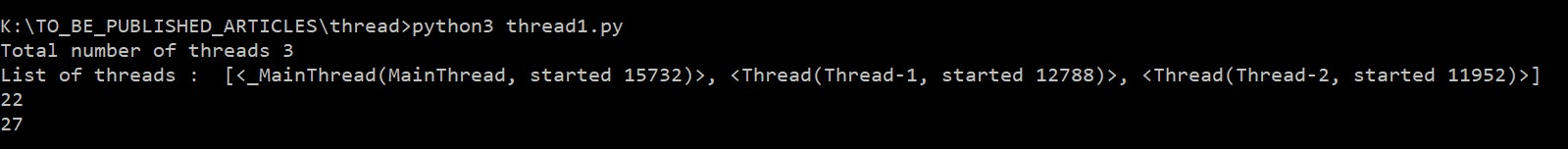Figure 1 : All threads and thread list

Now comment out the line `time.sleep(1)` and then run the code again.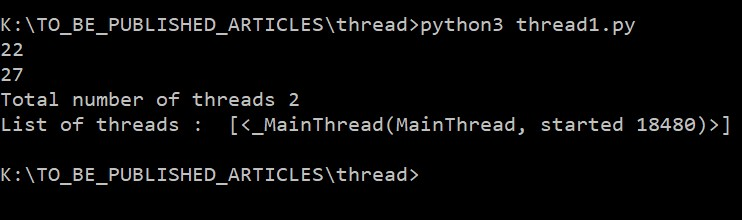Figure 2: Without sleep time

You can see the difference. The statement `threading.activeCount()` and `threading.enumerate()` have run the main thread or main process. So, the main thread is responsible for running the entire program. In Figure 1, when the main thread executed  `threading.activeCount()`, thread1 and thread2 were active.

When we commented out the `time.sleep(1)` syntax, and executed the code again then thread1 and thread3 might not be active.

## The Join Method

Before discussing the significance of the `join` method, let us see the following program.

In the above program, the two threads have been created with arguments. In the target function, `fun1`, a global list, `list1`, is appended with an argument. Let us see the result.

``````import threading

import time

list1 = []

def fun1(a):

time.sleep(1)# complex calculation takes 1 seconds

list1.append(a)

thread1 = threading.Thread(target = fun1, args = (1, ))

thread1.start()

thread2 = threading.Thread(target = fun1, args = (6, ))

thread2.start()

print(“List1 is: “, list1)``````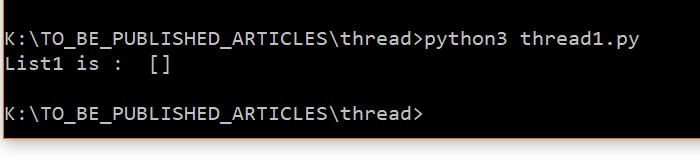Figure 3: Showing Empty list

The above figure is showing the empty list, but the list is expected to be filled with values 1 and 6. The statement `print (“List1 is : “, list1)` is executed by the main thread and the main thread printed the list before getting it filled.

The main thread, thus, must be paused until all threads complete their jobs. To achieve this, we shall use the `join` method.

Let's look at the modified code.

``````import threading

import time

list1 = []

def fun1(a):

time.sleep(1)# complex calculation takes 1 seconds

list1.append(a)

thread1 = threading.Thread(target = fun1, args = (1, ))

thread1.start()

thread2 = threading.Thread(target = fun1, args = (6, ))

thread2.start()

thread1.join()

thread2.join()

print(“List1 is: “, list1)``````

Ane here is the output.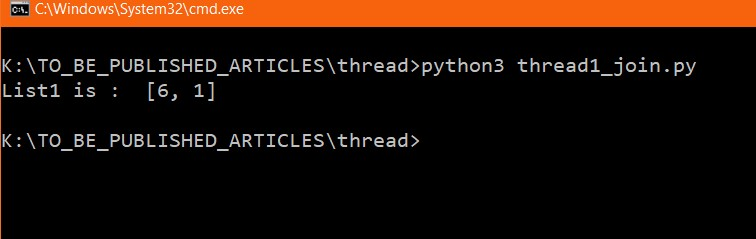Figure 4: Use of `join` method

In the above code, the syntax `thread1.join()` blocks the main thread until `thread1` finishes its task. In order to achieve parallelism, the `join` method must be called after the creation of all the threads.

Let's see more use cases of `join` methods.

``````import threading

import time

import datetime

t1 = datetime.datetime.now()

list1 = []

def fun1(a):

time.sleep(1)# complex calculation takes 1 seconds

list1.append(a)

list_thread = []

for each in range(10):

thread1 = threading.Thread(target = fun1, args = (each, ))

list_thread.append(thread1)

thread1.start()

for th in list_thread:

th.join()

print(“List1 is: “, list1)

t2 = datetime.datetime.now()

print(“Time taken”, t2 - t1)``````

In the above code, we have used a for loop to create 10 threads. Each thread is getting appended in the list, `list_thread`. After the creation of all the threads, we used the `join` method. Let's see the output.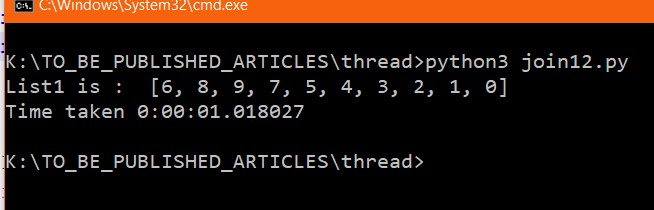Figure 5: Out of `join` with a loop

The time taken to execute is approximately 1 second. Since every thread takes 1 second and we used thread-based parallelism the total time taken to execute the code is close to 1 second. If you use the `join` method after the creation of each thread program it would take more than 10 seconds.

For more clarification, see the following code.

``````import threading

import time

import datetime

t1 = datetime.datetime.now()

list1 = []

def fun1(a):

time.sleep(1)# complex calculation takes 1 seconds

list1.append(a)

for each in range(10):

thread1 = threading.Thread(target = fun1, args = (each, ))

thread1.start()

thread1.join()

print(“List1 is: “, list1)

t2 = datetime.datetime.now()

print(“Time taken”, t2 - t1)``````

Output: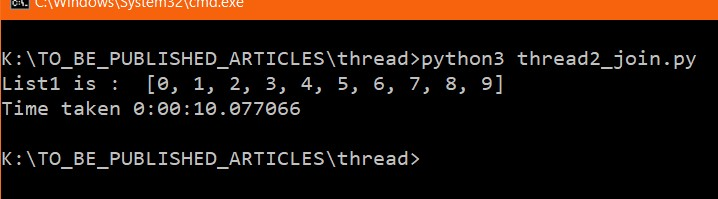Figure 6: Thread with `join` method

From the above output, it is clear that the time taken is 10 seconds. This means no parallelism has been achieved because the `join()` method of the first thread has been called before the creation of the second thread.

## join() Method With Time

Let us look at the following piece of code.

``````import threading

import time

def fun1(a):

time.sleep(3)# complex calculation takes 3 seconds

thread1 = threading.Thread(target = fun1, args = (1, ))

thread1.start()

thread1.join()

print(thread1.isAlive())``````

A couple of things are new here. The `isAlive()` method returns True or False. If the thread is currently active then the method `isAlive()` returns True; otherwise, it returns False.

Output: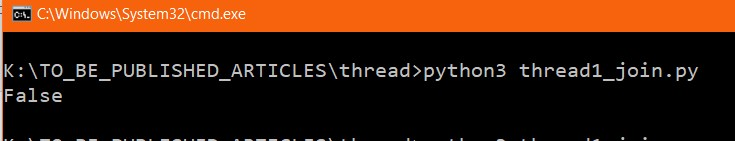The above output shows that the thread is not active.

Let's make a small change — change `thread1.join()` to `thread1.join(2)`. This tells the program to block the main thread only for 2 seconds.

Let's see the output: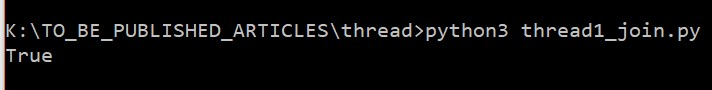In the above output, the thread was still active because `join(2)` kept blocking the main thread for 2 seconds but the thread took 3 seconds to complete its task.

## threading.Timer()

This method is used to set the time. Let us understand the syntax.

``threading.Timer(interval, function, args=[], kwargs={})``

The meaning of the above syntax is that after  a specified interval, in seconds, the interpreter will execute the function with `args` and `kwargs`.

Topics:
python ,python thread tutorial ,big data ,threading ,parallel execution

Comment (3)

Save
{{ articles.views | formatCount}} Views

Published at DZone with permission of

Opinions expressed by DZone contributors are their own.

# {{ parent.title || parent.header.title}}

{{ parent.tldr }}

{{ parent.urlSource.name }}